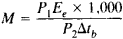# Ebullioscopy

Also found in: Dictionary, Medical, Wikipedia.
Related to Ebullioscopy: cryoscopy, Ebullioscopic constant

## Ebullioscopy

(also ebulliometry), a method of physicochemical analysis based on measuring the increase in the boiling point of a solution in comparison with the boiling point of a pure solvent.

According to Raoult’s laws (seeRAOULTS LAWS), for an infinitely diluted solution of a nonvolatile substance and in the absence of electrolytic dissociation, this increase, Δtb, is proportional to the molal concentration m of the solution; Δtb, = Eem, where Ee is the coefficient of proportionality (ebullioscopic constant), which is characteristic of each solvent and does not depend on the nature of the solute. Thus, Ee for water is equal to 0.526; for benzene, 2.57; for dioxane, 3.27; for camphor, 6.02; and for dibromoethane, 6.43; all quantities are expressed in °C. Along with cryoscopy, ebullioscopy is used to determine the molecular mass of a solute, the activity of the solvent and the solute, or the degree of dissociation of the weak electrolyte. The molecular mass M of the solute is computed according to the equationwhere P1, and P2 are the masses of the solute and the solvent, respectively, expressed in grams. The temperature difference Δtb is measured by means of a Beckmann thermometer or sensitive thermocouples.

### REFERENCE

Kurs fizicheskoi khimii, 2nd ed., vol. 1. Edited by Ia. I. Gerasimov. Moscow, 1969.

S. A. POGODIN

Site: Follow: Share:
Open / Close